• 分治法的另外一种排序算法，快速排序。有注释，便于阅读，因为交换时使用的引用，暂时归为C++，C语言版稍后奉上。
• 快速排序 快速排序是按照元素的值对他们进行划分。划分是对给定数组中的元素的重新排列,使得A[s]左边的元素都小于等于A[s],而所有A[s]右边的元素都大于等于A[s]。 A…A[s-1] A[s] A[s+1]…A[n-1] 建立了一个划分...
快速排序 快速排序是按照元素的值对他们进行划分。划分是对给定数组中的元素的重新排列,使得A[s]左边的元素都小于等于A[s],而所有A[s]右边的元素都大于等于A[s]。 A…A[s-1] A[s] A[s+1]…A[n-1] 建立了一个划分后，A[s]已经位于它在有序数组中的最终位置，接下来我们对A[s]前和A[s]后的子数组分别进行排序（使用同样的方法），注意在快速排序中，算法的主要工作在于划分阶段，而不需要再去合并子问题的解了 快速排序的划分算法 QuickSort(A[l…r]) //用QuickSort对子数组进行排序 //输入：数组A[0…n-1]中的子数组A[l…r],由左右下标l和r定义 //输出：非降序排列的子数组A[l…r] if l < r s <–GetStandard(A[l…r]) //s是每次分裂位置 QuickSort(A[l…s-1]) QuickSort(A[s+1…r]) 代码实现
void QuickSort(int a[], int low, int high) {     //开始默认基准为 low
if(low < high) {     //分段下表
printf("low  = %d, high = %d \n", low, high);
int standard = GetStandard(a, low, high);   //递归调用排序
QuickSort(a, low, standard - 1);   //左边排序
QuickSort(a, standard + 1, high);  //右边排序
}
}

快速排序的排序过程
int GetStandard(int a[], int i, int j){   //基准数据
int key = a[i];
while(i < j) {
while(i < j && a[j] >= key) {   //因为默认基准是从左边开始的，所以从右边开始比较
j--;             //当队尾的元素大于基准数据时，就一直向前挪动 j 指针
}
if(i < j) {
a[i] = a[j];    //当找到比 a[i] 小的时，就把后面的值 a[j] 赋值给它
}
while(i < j && a[i] <= key){
i++;    //当找到比 a[j] 大的时，就把前面的值 a[i] 赋值给它
}
if(i < j) {
a[j] = a[i];
}
}               //跳出循环时，i 和 j 的值相等，此时 i 或 j 就是 key 的正确索引位置
a[i] = key;    //把基数数据赋给正确位置
return i;
}

例题 用分治法对 5, 3, 1, 9, 8, 2, 4, 7 进行快速排序
#include<stdio.h>
int GetStandard(int a[], int i, int j){   //基准数据
int key = a[i];
while(i < j) {
while(i < j && a[j] >= key) {   //因为默认基准是从左边开始的，所以从右边开始比较
j--;             //当队尾的元素大于基准数据时，就一直向前挪动 j 指针
}
if(i < j) {
a[i] = a[j];    //当找到比 a[i] 小的时，就把后面的值 a[j] 赋值给它
}
while(i < j && a[i] <= key){
i++;    //当找到比 a[j] 大的时，就把前面的值 a[i] 赋值给它
}
if(i < j) {
a[j] = a[i];
}
}               //跳出循环时，i 和 j 的值相等，此时 i 或 j 就是 key 的正确索引位置
a[i] = key;    //把基数数据赋给正确位置
return i;
}

void QuickSort(int a[], int low, int high) {     //开始默认基准为 low
if(low < high) {     //分段下表
printf("low  = %d, high = %d \n", low, high);
int standard = GetStandard(a, low, high);   //递归调用排序
QuickSort(a, low, standard - 1);   //左边排序
QuickSort(a, standard + 1, high);  //右边排序
}
}

void printf(int a[], int n){
for(int i = 0; i < n; i++)
printf("%-3d", a[i]);
}

int main(){
int a = {5, 3, 1, 9, 8, 2, 4, 7};
for(int i = 0; i < 8; i++)
printf("%-3d", a[i]);
printf("\n");
QuickSort(a, 0, 7);
printf(a, 8);
return 0;
}

运行结果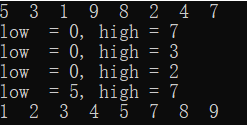代码分析 利用递归调用树，分析递归调用的过程，调入值是子数字的边界 l 和 r 以及划分的分裂位置 s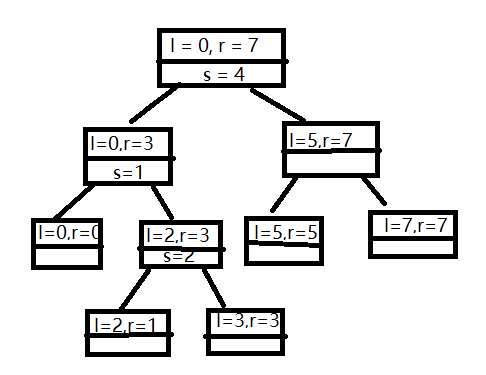时间复杂度：nlogn 快速排序是不稳定算法
结尾 写博客是为了一是整理所学知识，亲生写代码的经验，而是为了总结经典算法，三是督促自己努力，懂得越多，越知道自己知识的浅薄，四是希望和他人多多交流，有什么不对的地方大佬们多多指点
展开全文算法
• 主要介绍了Java基于分治法实现的快速排序算法,结合实例形式分析了java基于分治法快速排序相关实现技巧,代码中备有较为详细的注释说明便于理解,需要的朋友可以参考下
• 这个代码是利用快速排序算法，求第K大的数。 快速排序由C. A. R. Hoare在1960年提出。它的基本思想是：通过一趟排序将要排序的数据分割成独立的两部分，其中一部分的所有数据都比另外一部分的所有数据都要小，然后...
• 分治法 - 快速排序 题目描述 利用快速排序算法将读入的 N个数从小到大排序后输出，请勿使用std::sort。 输入格式 第一行一个整数 n (1≤n≤105)。 第二行 n 个整数 ai (1≤ai≤109)。 输出格式 输出一行，为 ai 排序...
分治法 - 快速排序
题目描述
利用快速排序算法将读入的 N个数从小到大排序后输出，请勿使用std::sort。
输入格式
第一行一个整数 n (1≤n≤105)。
第二行 n 个整数 ai (1≤ai≤109)。
输出格式
输出一行，为 ai 排序后的结果。
解题思路
先从数列中取出一个基准数，将比这个数大的数全放在它右边，小于这个数的数全放在左边，再对左右区间重复，直到每个区间只有一个数。
当left<right时，才开始。以temp为基准，temp=a[left],i=left,j=right
若a[j]>=temp,j-- ,从右往左扫描
若a[i]<=temp,i++,从左往右扫描
交换a[i]、a[j].
出循环后，再改变基准，然后对左右区间分别递归调用
完整代码及测试结果
#include<stdio.h>
void quicksort(int left,int right,int a[]){
int i,j,temp,k;
if(left>right){
return;
}
temp = a[left];
i = left;
j = right;
while(i<j){
while(a[j]>=temp && i<j)j--;

while(a[i]<=temp && i<j)i++;

if(i<j){
k = a[i];
a[i] = a[j];
a[j] = k;
}
}
a[left] = a[i];
a[i] = temp;
quicksort(left,i-1,a);
quicksort(i+1,right,a);

}

int main(){
int a;
int i=0,n;
scanf("%d",&n);
getchar();
char temp;
while(i<n)
{
scanf("%d",&a[i]);
getchar();
i++;
}
quicksort(0,i-1,a);
int j;
for(j=0;j<i;j++){
printf("%d ",a[j]);
}
return 0;
}

一组测试用例及结果展开全文算法 排序算法
• 分治法的应用，快速排序是其中一种。注释便于读者明白。
• 课程的随堂作业，C语言的，用dev就能运行，萌新代码，勿喷，仅仅帮助不想写作业的朋友方便一下，反正老师也不会仔细检查的
• //进行一趟排序，返回枢纽位置 //a[low]=a int pivotkey=a[low];//保存枢纽记录关键字，用子表的第一个记录做枢纽记录 while(low) //从序列两端交替向中间扫描，直到low=high为止 { while(low[high]...
手写快排比Java自带的Arrays.sort()快吗？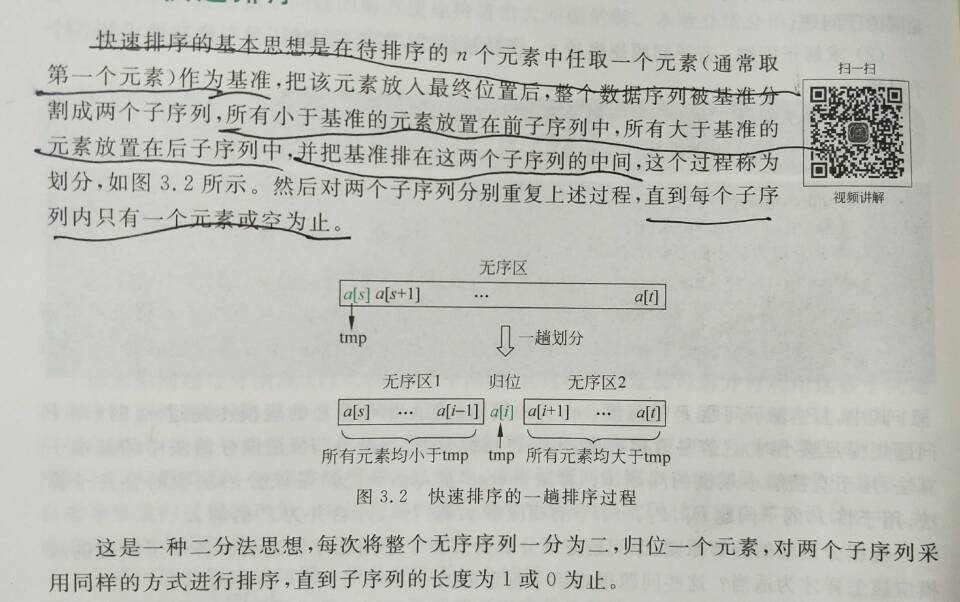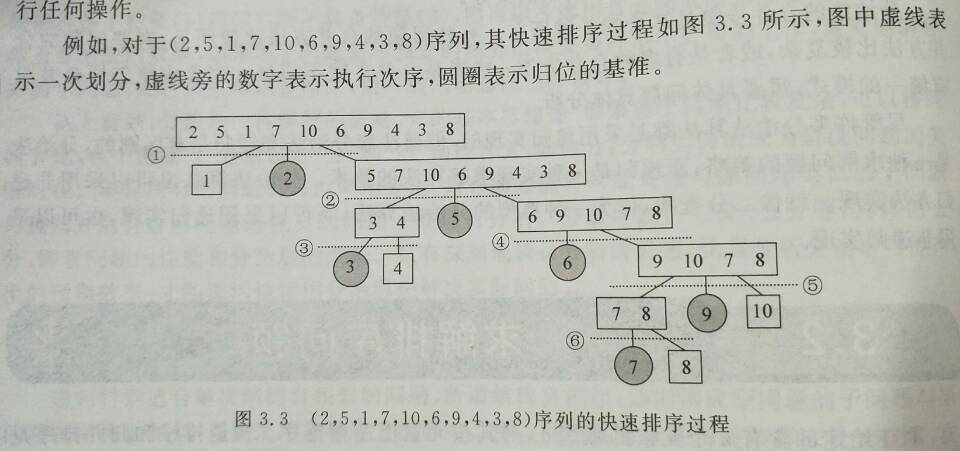代码如下：
public class quick_sort {
//输出函数
static void disp(int[] a,int  n){
int i;
for(i=0;i<n;i++){
System.out.print(a[i]+" ");
}
System.out.println();
}

//划分算法
static int partition(int a[],int low,int high)//partition 划分，分割，分区
{
//进行一趟排序，返回枢纽位置
//a[low]=a
int pivotkey=a[low];//保存枢纽记录关键字，用子表的第一个记录做枢纽记录
while(low<high) //从序列两端交替向中间扫描，直到low=high为止
{
while(low<high && a[high]>=pivotkey)//从右向左寻找，直到找到一个比枢纽小的元素
high--;
if(low<high)
a[low]=a[high];//比枢纽小的记录移到低端
while(low<high && a[low]<=pivotkey) //从左往右寻找，直到找到一个比枢纽大的元素
low++;
if(low<high)
a[high]=a[low];//比枢纽大的记录移到高端
}
a[low]=pivotkey;//枢纽记录到位
return low;//返回枢纽位置
}

//递归排序
static void quickSort(int a[],int low,int high){
if(low<high){
int pivotloc=partition(a,low,high); //pivotloc是枢纽位置
quickSort(a,low,pivotloc-1);  //对左子表递归排序
quickSort(a,pivotloc+1,high);  //对右子表递归排序
}
}
public static void main(String[] args) {
// TODO 自动生成的方法存根
int n=10;
int[] a={2,5,1,7,10,6,9,4,3,8};
System.out.print("排序前：");
disp(a,n);
System.out.print("排序后：");
quickSort(a, 0, n-1);
disp(a,n);

}

}

展开全文• 分治法的基本思想是将一个规模为N的问题分解为K个规模较小的子问题，这些子问题相互独立且与原问题性质相同。求出子问题的解，就可得到原问题的解。即一种分目标完成程序算法，简单问题可用二分法完成。 分治法的...
分治算法
分治法的基本思想是将一个规模为N的问题分解为K个规模较小的子问题，这些子问题相互独立且与原问题性质相同。求出子问题的解，就可得到原问题的解。即一种分目标完成程序算法，简单问题可用二分法完成。
分治法的精髓
分--将问题分解为规模更小的子问题；
治--将这些规模更小的子问题逐个击破；
合--将已解决的子问题合并，最终得出“母”问题的解。
应用
分治法具体应用有查找技术还有排序技术。查找技术有二分查找;排序有快速排序还有归并排序等。
排序:
快速排序、归并排序。
快速排序:
快速排序应该场景:数据量大并且是线性结构。
短处:有大量重复数据的时候，性能不好单向链式结构处理性能不好（一般来说，链式都不使用）。
步骤:
1.取出数组中最前的数据作为对比数据;定义低位指针和高位指针,分别是数组头部第一个数据和尾部第一个数据。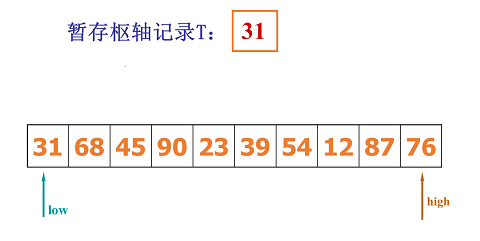2.(1)如上图所示,我们需要右移低位指针和左移高位指针取出数据,先取出76>31,高位指针向左移动,低位指针保持不动
(2)当高位指针移动到12位置时,12<31,将会发生交换,取出12与低位指针上的数据进行交换,如下图: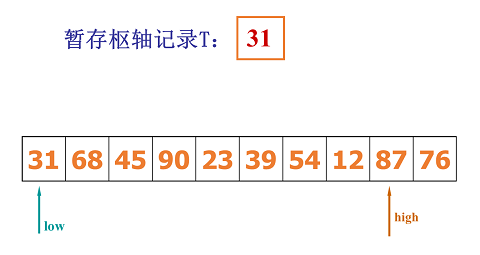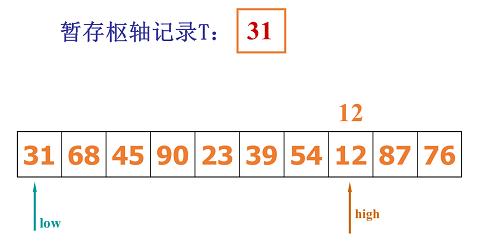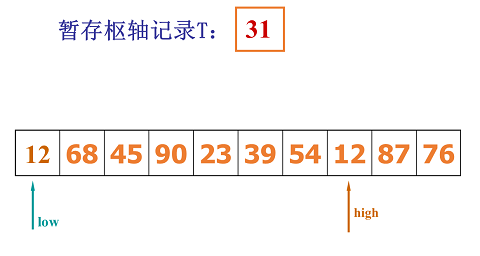3.(1)当发生数据交换后,低位指针要开始变化,通过低位指针右移一位到68进行对比,68>31,将取出68与高位指针数据进行交换
(2)注意:如果取出的数小于31,将继续右移低位指针,进行数据对比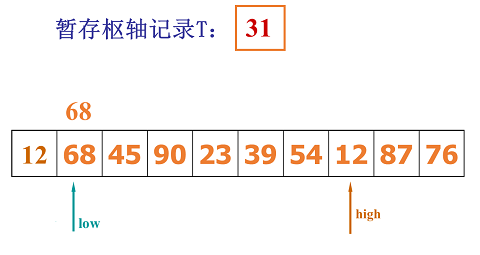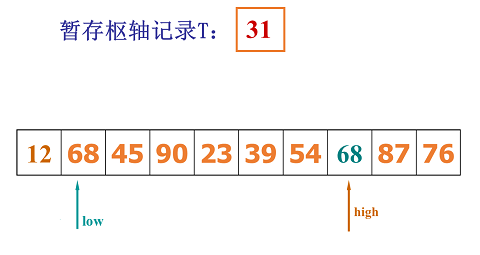4.进行完数据交换后,继续左移高位指针,继续将高位指针上的数据进行对比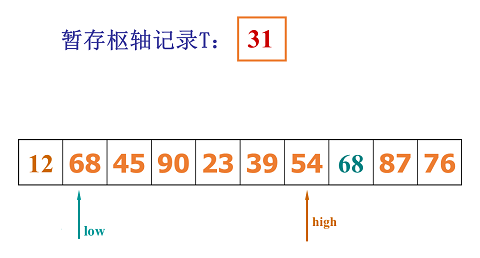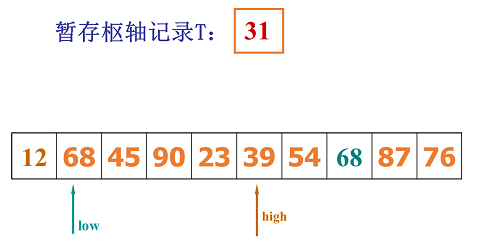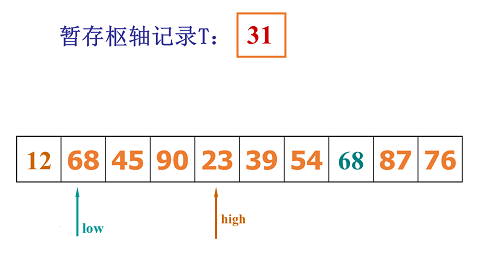4.当发现23<31时,继续重复以上步骤2、步骤3,进行数据交换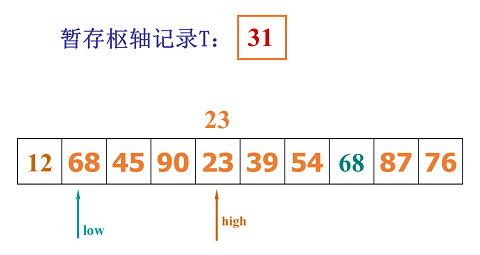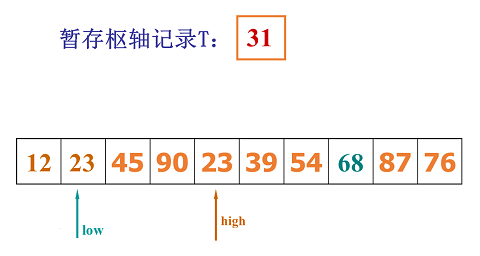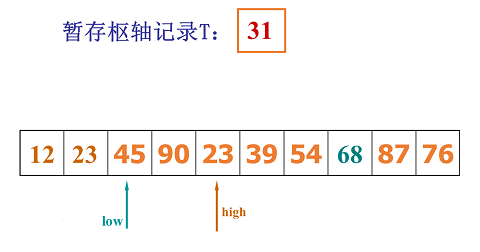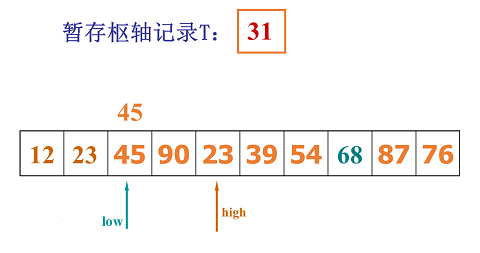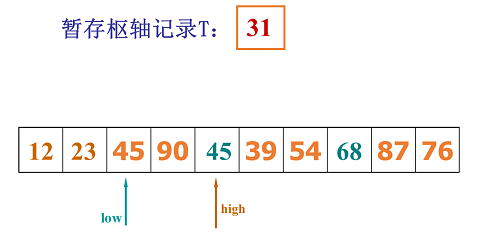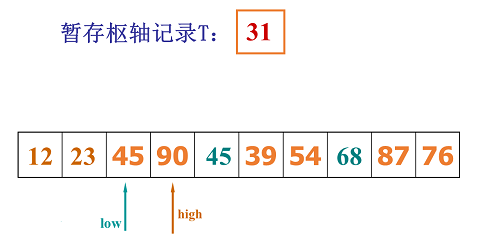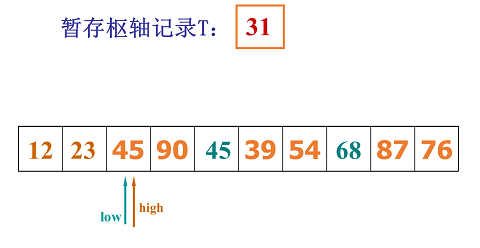5.当最后高位指针和低位指针重合时,将31合并到该位置。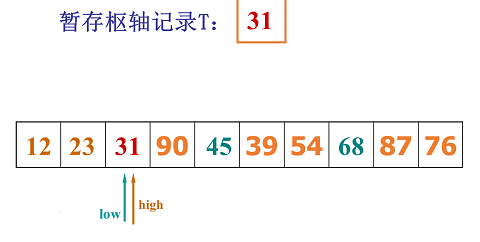6.以上就是快速排序的一次分隔过程。接下来,用代码实现过程。
/**
* 快速排序
* @param array
* @param begin
* @param end
*/
public static void quickSort(int[] array,int begin,int end){
if(end-begin<=0) return;
int x=array[begin];
int low=begin;
int high=end;
boolean direction=true;  //标记方向,高低位指针需要左移右移
L1:
while(low<high){
if(direction){      //从右往左找
for(int i=high;i>low;i--){
if(array[i]<=x){
array[low++]=array[i];
high=i;
direction=!direction;
continue L1;
}
}
high=low;//未比较大小,两个指针重合
}else{
for(int i=low;i<high;i++){
if(array[i]>=x){
array[high--]=array[i];
low=i;
direction=!direction;
continue L1;
}
}
low=high;
}
}
array[low]=x;   //把最后找到的值,放入中间位置
//以上只是进行一次分隔,所以接下来,我们需要再对左右两端进行分隔操作
quickSort(array,begin,low-1);
quickSort(array,low+1,end);
}

展开全文• 2.6 快速排序(quick sort) 2.6.1 算法思路 **整体思路：**随机选一个数，把比它大的数放在它的右边，小的放在左边，那个数所在的位置，就会是它最终排序所在的位置。然后继续排左右两边的数，按这种思路往下递归。 *...递归 时间复杂度
• 使用快速排序算法实现对n个元素进行排序。 由文件input.txt提供输入数据，输出到文件output.txt中。
• 分治法 快速排序 快速排序动画演示 def quicksort(arr,left = None,right = None): #快速排序 arr-数列，lerf-数列最左元素下标，right-数列最右元素下标 left = 0 if not isinstance(left,int) else left #左下标...算法 python
• 分治法Q3——快速排序 package 分治; public class 快速排序 { /**快速排序法 分治实现 * 重点在分区根据分区方法不同分为：单向扫描法 双向扫描法 * template:1.terminator 2.process(prepare data,split ...算法 排序算法
• 方法:分治法求解问题的思想是将问题中通过整体代入的思想将问题递归地分成小问题（即规模较小的原问题），并且每一次递归后都要进行合并操作，只有这样在递归结束后，分解的小问题的解就自动合并成最终的解...quickSort
• ## 分治法-快速排序

千次阅读 多人点赞 2017-12-18 20:38:32
快速排序由C. A. R. Hoare在1962年提出。它的基本思想是：通过一趟排序将要排序的数据分割成独立的两部分，其中一部分的所有数据都比另外一部分的所有数据都要小，然后再按此方法对这两部分数据分别进行快速排序，...
• 用c++实现的快速排序的源代码如下： #include <iostream> #include <cstdlib> using namespace std; //划分算法 int Partition(int a[],int l,int r) { int pilot = a[l]; //用序列的第一个记录作为...算法 排序算法
• 快速排序算法的基本思想是：先找一个基准元素（比如待排序数组的第一个元素），进行一趟快速排序，使得该基准元素左边的所有数据都它小，而右边的所有数据都它大，然后再按此方法，对左右两边的数据分别进行快速排序...算法思路
• #C语言七大排序算法之分治 在上个星期，我看完了七大排序算法（其实是看完了除选择、冒泡、插入排序之外的四种） 而在这篇文章中，我会把...快速排序算法 归并排序算法 快速排序算法到底有多快呢（来看这张表吧） ...
• 分治法-快速排序-java语言实现 问题描述: 输入一个数字N后,输入N个数字,将N个数字排序后输出. 输入: 8 1 6 5 2 3 8 7 9 输出: 1 2 3 5 6 7 8 9算法
• 快速排序： 初始键值序列： 34 20 71 26 23 9 44 35 i j 右侧扫描直到R[j]<34,且交换后i++： 9 20 71 26 23 34 44 35 i j 左侧扫描直到R[i]>34,且交换后j–： 9 20 34 26 23 71 44 35 i j 右侧扫描直到...
• 目录算法设计思想典型例题一、归并排序运行结果二、快速排序 算法设计思想 分治法字面上理解就是“分而治之”，把一个复杂的问题分解成两个或者多个相似的问题，再把子问题分成更小的子问题，直到子问题可以简单的...算法 排序算法
• def partition(seq): pi,seq = seq,seq[1:] lo = [x for x in seq if x <= pi] hi = [x for x in seq if x > pi] return lo,pi,hi def quicksort(seq): if len(seq) <= 1: ...
• 今天，再次分享分治法的第三个经典算法：快速排序（含随机快速排序） 需要的朋友请自取哦~ 欢迎大家与我一起交流呀~~ 一起学习，一起进步！ < public class quickSort { static Comparable[] a;//待排数组 //...
• 是一种分治法，通过大问题划分为小问题 该方法的基本思想是： 1．先从数列中取出一个数作为基准数。 2．分区过程，将比这个数大的数全放到它的右边，小于或等于它的数全放到它的左边。 3．再对左右区间重复第二...排序算法 数据结构
• 快速排序 基本思想： 将首元素x作为划分标准，将输入数组A划分为不超过x的元素构成数组A1，将大于x的元素构成的数组作为A2，从左到右存放在数组A的位置。 递归的对子问题A1,A2进行排序，知道子问题规模为1时停止...c
• title: 快速排序法（quick sort） tags: 分治法（divide and conquer method） grammar_cjkRuby: true --- 算法原理 分治法的基本思想：将原问题分解为若干个更小的与原问题相似的问题，然后递归解决各个子问题，...
• 一、快速排序 （1）基本思想 在待排序的n个元素中任取一个元素（通常取第一个元素）作为基准，把该元素放入最终位置后，整个数据序列被基准值分割成两个子序列，所有小于基准值的元素放置在前子序列中，所有大于...
• ## 分治法_快速排序

千次阅读 2017-03-25 15:45:54
分治法的思想：将大的问题化为小问题；问题性质不变  快速排序是在比较排序中相对较快，所以称为快速排序。对于一个数组a[n]快速排序中分界值需要取n次也就是说，每一个下标对应的值都需要取一次。  对于...c++ 排序...